# Selina Concise Mathematics Class 8 ICSE Solutions Chapter 6 Sets

## Selina Concise Mathematics Class 8 ICSE Solutions Chapter 6 Sets

Selina Publishers Concise Mathematics Class 8 ICSE Solutions Chapter 6 Sets

### Sets Exercise 6A – Selina Concise Mathematics Class 8 ICSE Solutions

Question 1.
Write the following sets in roster (Tabular) form :
(i) A1 = {x : 2x + 3 = 11}
(ii) A2 = {x : x2 – 4x – 5 = 0}
(iii) A3 = {x : x ∈ Z, -3 ≤ x < 4}
(iv) A4 = {x : x is a two digit number and sum of digits of x is 7}
(v) A5 = {x : x = 4n, n ∈ W and n < 4}
(vi) A6 = {x : x = $$\frac{n}{n+2}$$; n ∈ N and n > 5}
Solution: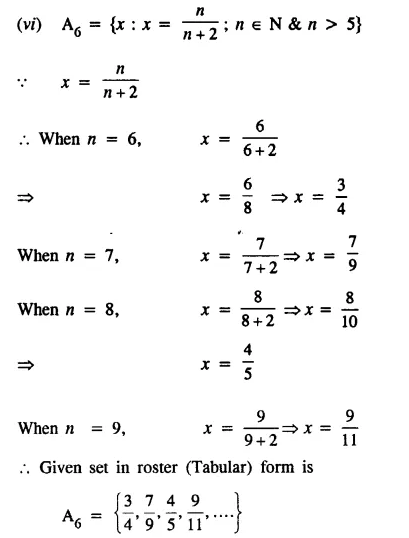Question 2.
Write the following sets in set-builder (Rule Method) form :Solution:Question 3.
(i) Is {1, 2, 4, 16, 64} = {x : x is a factor of 32} ? Give reason.
(ii) Is {x : x is a factor of 27} ≠ {3, 9, 27, 54} ? Give reason.
(iii) Write the set of even factors of 124.
(iv) Write the set of odd factors of 72.
(v) Write the set of prime factors of 3234.
(vi) Is {x : x2 – 7x + 12 = 0} = {3, 4} ?
(vii) Is {x : x2 – 5x – 6 = 0} = {2, 3} ?
Solution: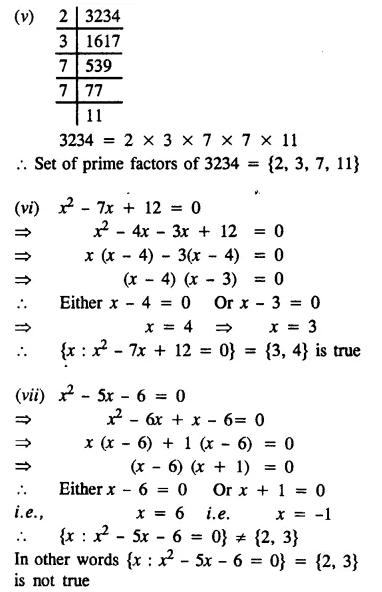Question 4.
Write the following sets in Roster form :
(i) The set of letters in the word ‘MEERUT’.
(ii) The set of letters in the word ‘UNIVERSAL’.
(iii) A = {x : x = y + 3, y ∈N and y > 3}
(iv) B = {p : p ∈ W and p2 < 20}
(v) C = {x : x is composite number and 5 < x < 21}
Solution: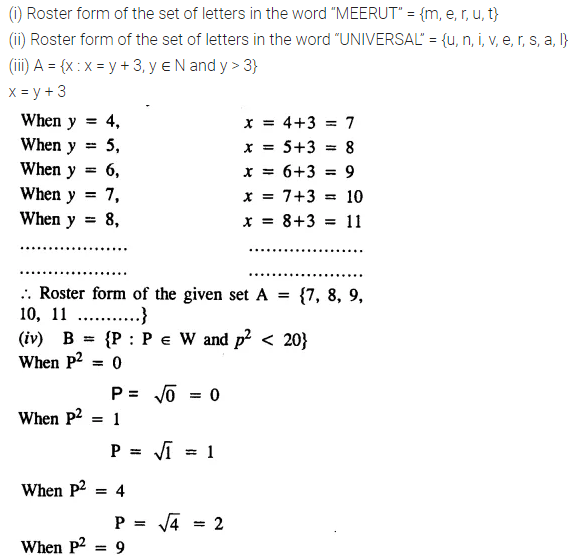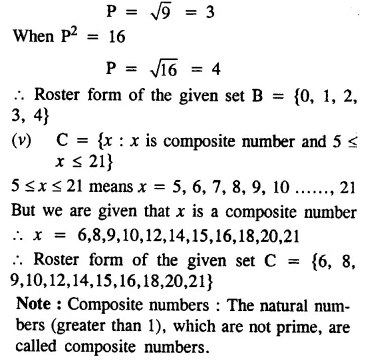Question 5.
List the elements of the following sets :
(i) {x : x2 – 2x – 3 = 0}
(ii) {x : x = 2y + 5; y ∈ N and 2 ≤ y < 6}
(iii) {x : x is a factor of 24}
(iv) {x : x ∈ Z and x2 ≤ 4}
(v) {x : 3x – 2 ≤ 10, x ∈ N}
(vi) {x : 4 – 2x > -6, x ∈ Z}
Solution: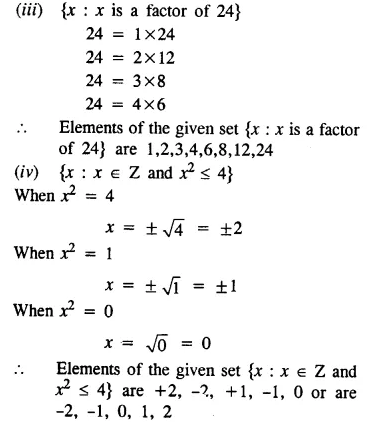### Sets Exercise 6B – Selina Concise Mathematics Class 8 ICSE Solutions

Question 1.
Find the cardinal number of the following sets :
(i) A1 = {-2, -1, 1, 3, 5}
(ii) A2 = {x : x ∈ N and 3 ≤ x < 7}
(iii) A3 = {p : p ∈ W and 2p – 3 < 8}
(iv) A4 = {b : b ∈ Z and -7 < 3b – 1 ≤ 2}
Cardinal Number of a set; The number of elements in a set is called is Cardinal Number.
Solution:Question 2.
If P = {P : P is a letter in the word “PERMANENT”}. Find n (P).
Solution: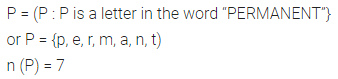Question 3.
State, which of the following sets are finite and which are infinite :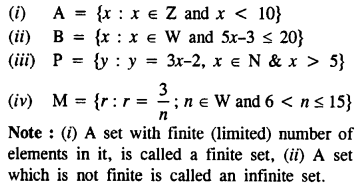Solution: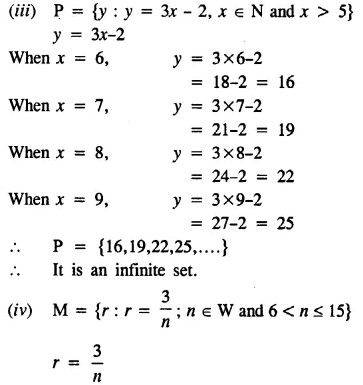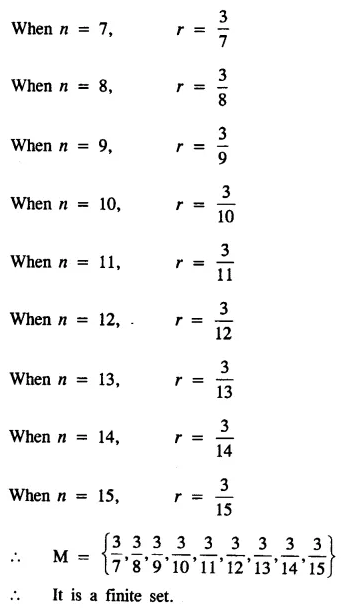Question 4.
Find, which of the following sets are singleton sets :
(i) The set of points of intersection of two non-parallel st. lines in the same plane
(ii) A = {x : 7x – 3 = 11}
(iii) B = {y : 2y + 1 < 3 and y ∈ W}
Note : A set, which has only one element in it, is called a SINGLETON or unit set.
Solution: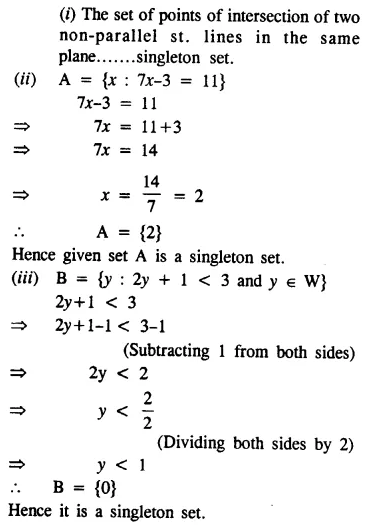Question 5.
Find, which of the following sets are empty :
(i) The set of points of intersection of two parallel lines.
(ii) A = {x : x ∈ N and 5 < x < 6}
(iii) B = {x : x2 + 4 = 0, x ∈ N}
(iv) C = {even numbers between 6 & 10}
(v) D = {prime numbers between 7 & 11}
Note : The set, which has no element in it, is called the empty or null set.
Solution: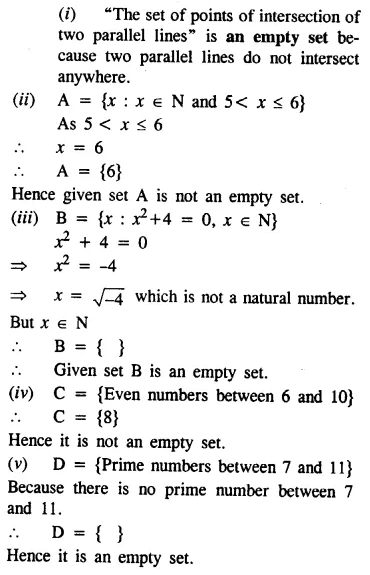Question 6.
(i) Are the sets A = {4, 5, 6} and B = {x : x2 – 5x – 6 = 0} disjoint ?
(ii) Are the sets A = {b, c, d, e} and B = {x : x is a letter in the word ‘MASTER’} joint ?
Note :
(i) Two sets are said to be joint sets, if they have atleast one element in common.
(ii) Two sets are said to be disjoint, if they have no element in common.
Solution: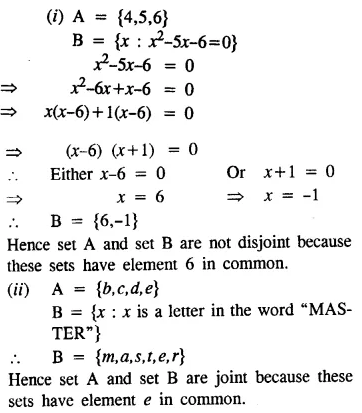Question 7.
State, whether the following pairs of sets are equivalent or not :
(i) A = {x : x ∈ N and 11 ≥ 2x – 1} and B = {y : y ∈ W and 3 ≤ y ≤ 9}
(ii) Set of integers and set of natural numbers.
(iii) Set of whole numbers and set of multiples of 3.
(iv) P = {5, 6, 7, 8} and M = {x : x ∈ W and x < 4}
Note : Two sets are said to be equivalent, if they contain the same number of elements.
Solution: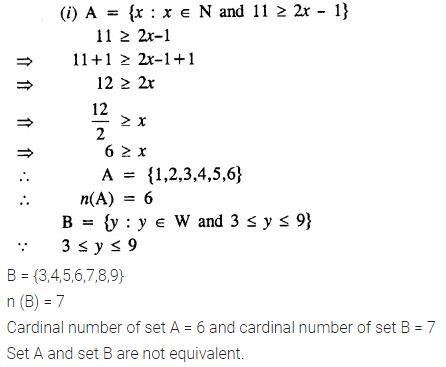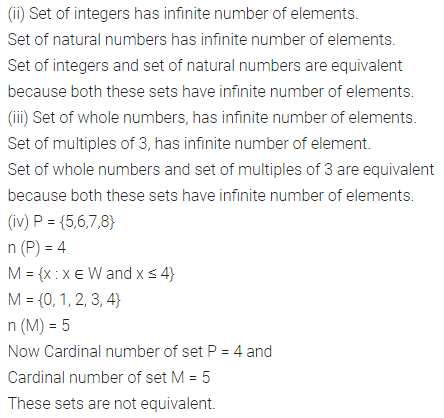Question 8.
State, whether the following pairs of sets are equal or not :
(i) A = {2, 4, 6, 8} and
B = {2n : n ∈ N and n < 5}
(ii) M = {x : x ∈ W and x + 3 < 8} and
N = {y : y = 2n – 1, n ∈ N and n < 5}
(iii) E – {x : x2 + 8x – 9 = 0} and
F = {1, -9}
(iv) A = {x : x ∈ n, x < 3} and
B = {y : y2 – 3y + 2 = 0}
Note: Two sets are equal, if both the sets have same (identical) elements.
Solution: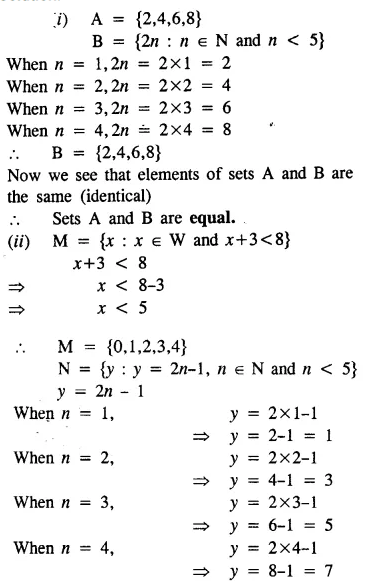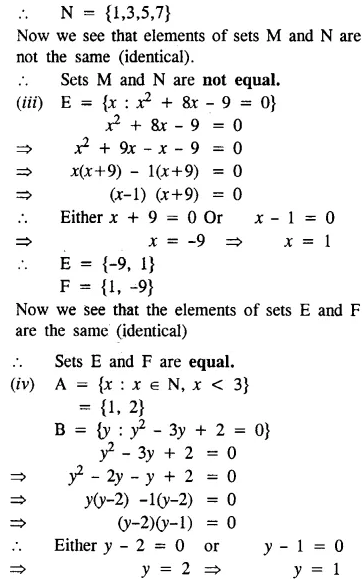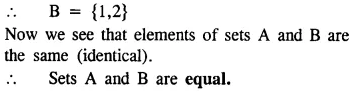Question 9.
State whether each of the following sets is a finite set or an infinite set:
(i) The set of multiples of 8.
(ii) The set of integers less than 10.
(iii) The set of whole numbers less than 12.
(iv) {x : x = 3n – 2, n ∈ W, n ≤ 8}
(v) {x : x = 3n – 2,n ∈ Z, n ≤ 8}
(vi) {x : x = $$\frac { n-2 }{ n+1 }$$ , n ∈ w)
Solution: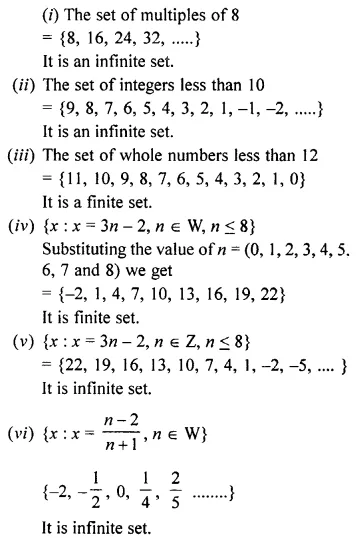Question 10.
Answer, whether the following statements are true or false. Give reasons.
(i) The set of even natural numbers less than 21 and the set of odd natural numbers less than 21 are equivalent sets.
(ii) If E = {factors of 16} and F = {factors of 20}, then E = F.
(iii) The set A = {integers less than 20} is a finite set.
(iv) If A = {x : x is an even prime number}, then set A is empty.
(v) The set of odd prime numbers is the empty set.
(vi) The set of squares of integers and the set of whole numbers are equal sets.
(vii) In n(P) = n(M), then P → M.
(viii) If set P = set M, then n(P) = n(M).
(ix) n(A) = n(B) ⇒ A = B.
Solution: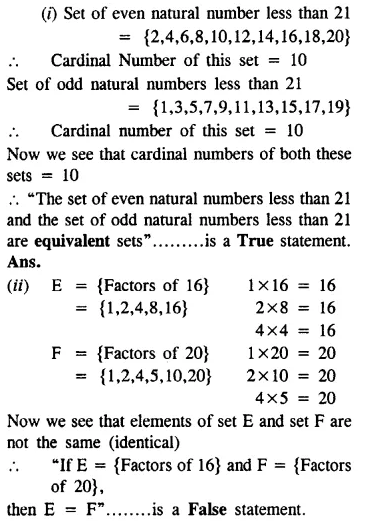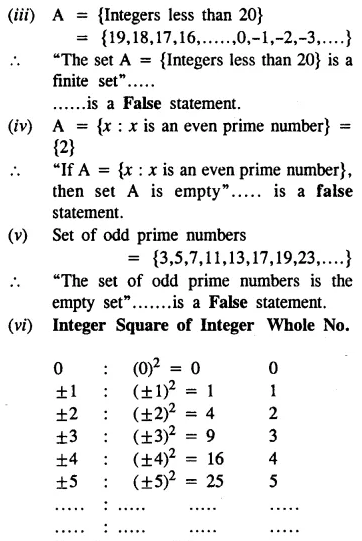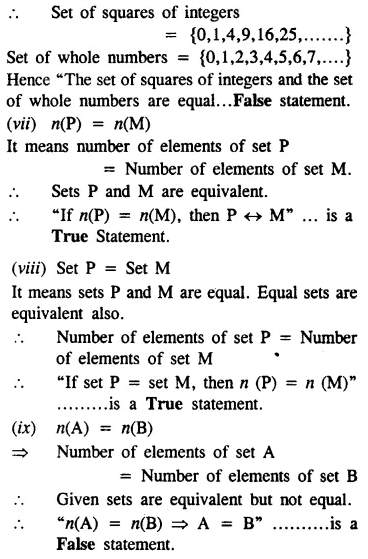### Sets Exercise 6C – Selina Concise Mathematics Class 8 ICSE Solutions

Question 1.
Find all the subsets of each of the following sets :
(i) A = {5, 7}
(ii) B = {a, b, c}
(iii) C = {x : x ∈ W, x ≤ 2}
(iv) {p : p is a letter in the word ‘poor’}
Solution:Question 2.
If C is the set of letters in the word “cooler”, find :
(i) Set C
(ii) n(C)
(iii) Number of its subsets
(iv) Number of its proper subsets.
Note : (i) If a set has n elements, the number of its subsets = 2n
(ii) If a set has n elements, the number of its proper subsets = 2n – 1
Solution:Question 3.
If T = {x : x is a letter in the word ‘TEETH’}, find all its subsets.
Solution: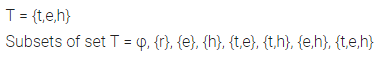Question 4.
Given the universal set = {-7,-3, -1, 0, 5, 6, 8, 9}, find :
(i) A = {x : x < 2}
(ii) B = {x : -4 < x < 6}
Solution: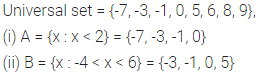Question 5.
Given the universal set = {x : x ∈ N and x < 20}, find :
(i) A = {x : x = 3p ; p ∈ N}
(ii) B = {y : y – 2n + 3, n ∈ N}
(iii) C = {x : x is divisible by 4}
Solution:Question 6.
Find the proper subsets of {x : x2 – 9x – 10 = 0}
Solution:Question 7.
Given, A = {Triangles}, B = {Isosceles triangles}, C = {Equilateral triangles}. State whether the following are true or false. Give reasons.
(i) A ⊆ B
(ii) B ⊆ A
(iii) C ⊆  B
(iv) B ⊂ A
(v) C ⊂ A
(vi) C ⊆  B ⊆  A
Solution: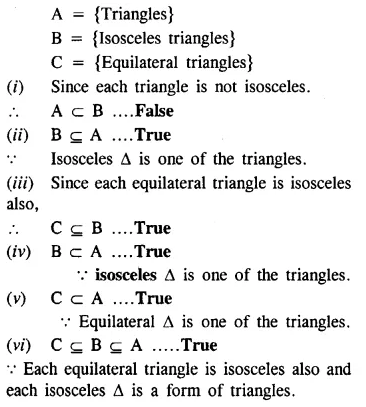Question 8.
Given, A = {Quadrilaterals}, B = {Rectangles}, C = {Squares}, D= {Rhombuses}. State, giving reasons, whether the following are true or false.
(i) B ⊂ C
(ii) D ⊂ B
(iii) C ⊆ B ⊆ A
(iv) D ⊂ A
(v) B ⊇ C
(vi) A ⊇ B ⊇ D
Solution:Question 9.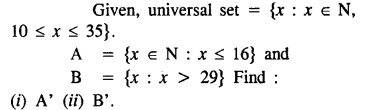Solution:Question 10.Solution:Question 11.Solution:Question 12.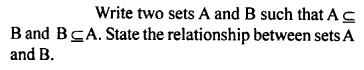Solution: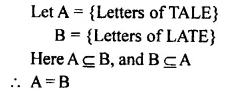### Sets Exercise 6D – Selina Concise Mathematics Class 8 ICSE Solutions

Question 1.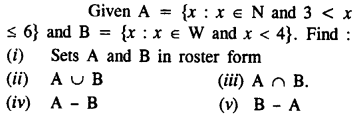Solution:Question 2.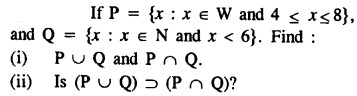Solution:Question 3.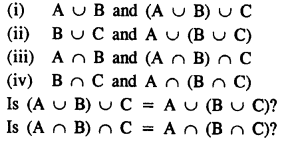Solution:Question 4.Solution:Question 5.Solution:Question 6.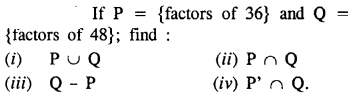Solution: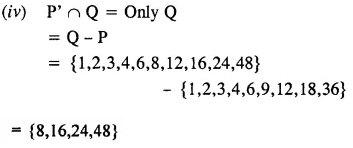Question 7.Solution: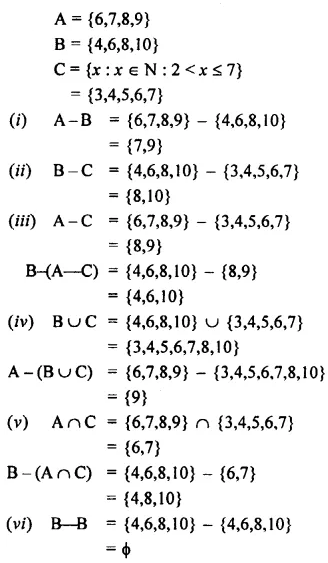Question 8.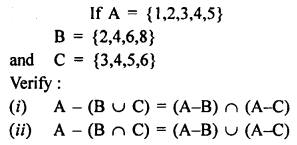Solution:Question 9.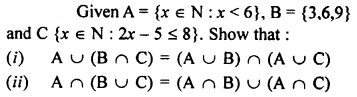Solution: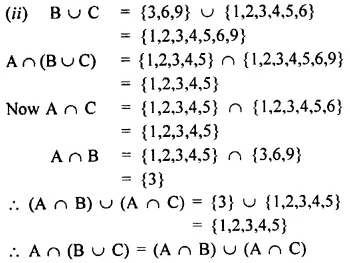### Sets Exercise 6E – Selina Concise Mathematics Class 8 ICSE Solutions

Question 1.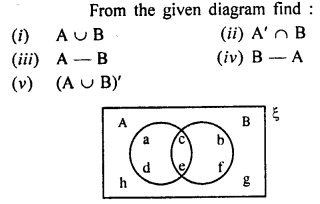Solution: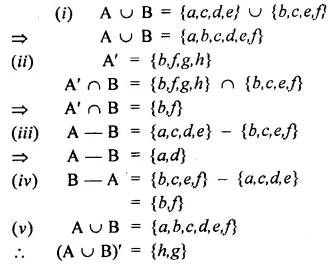Question 2.
From the given diagram, find :
(i) A’
(ii) B’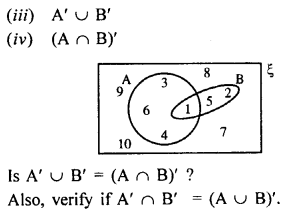Solution:Question 3.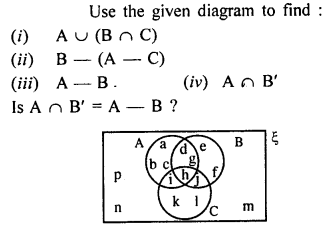Solution: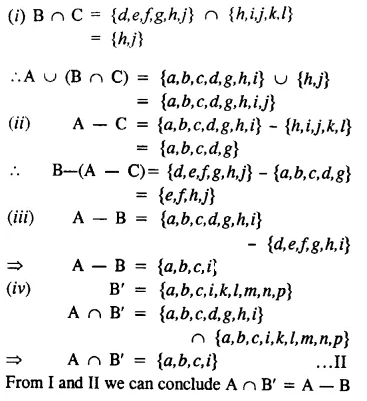Question 4.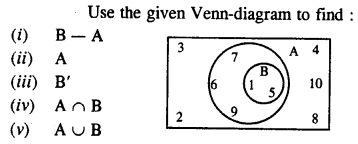Solution:Question 5.Solution:Question 6.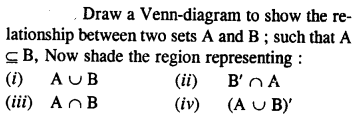Solution: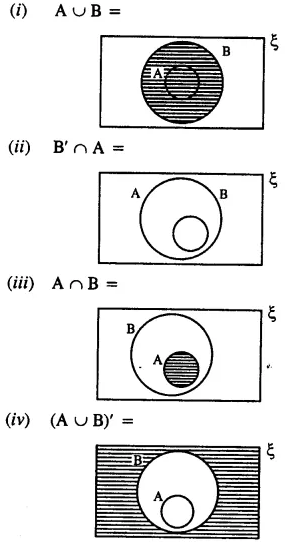Question 7.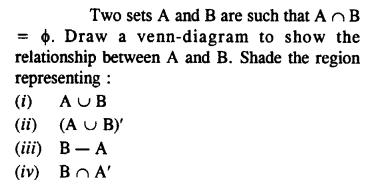Solution:Question 8.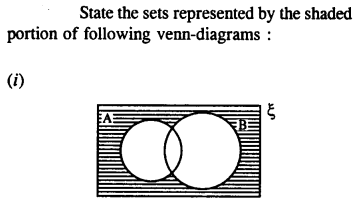Solution:Question 9.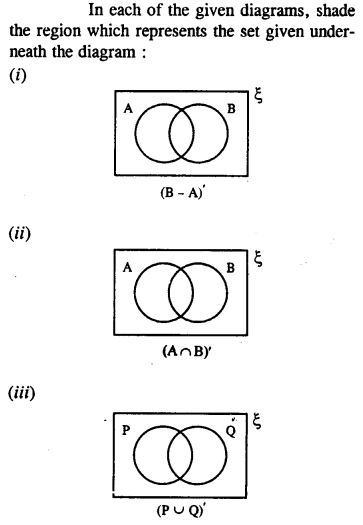Solution: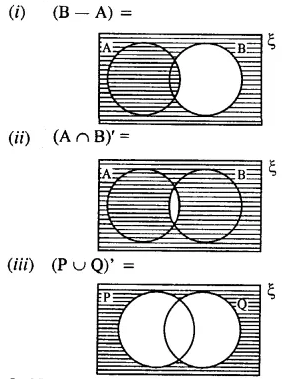Question 10.Solution: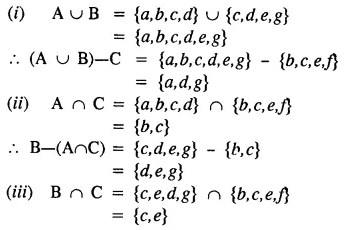Question 11.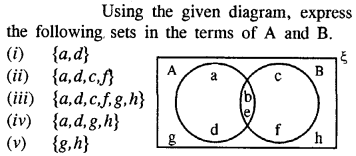Solution: